NEET  >  31 Year NEET Previous Year Questions: Electrostatics Potential & Capacitance

# 31 Year NEET Previous Year Questions: Electrostatics Potential & Capacitance

Test Description

## 36 Questions MCQ Test Physics Class 12 | 31 Year NEET Previous Year Questions: Electrostatics Potential & Capacitance

31 Year NEET Previous Year Questions: Electrostatics Potential & Capacitance for NEET 2023 is part of Physics Class 12 preparation. The 31 Year NEET Previous Year Questions: Electrostatics Potential & Capacitance questions and answers have been prepared according to the NEET exam syllabus.The 31 Year NEET Previous Year Questions: Electrostatics Potential & Capacitance MCQs are made for NEET 2023 Exam. Find important definitions, questions, notes, meanings, examples, exercises, MCQs and online tests for 31 Year NEET Previous Year Questions: Electrostatics Potential & Capacitance below.
Solutions of 31 Year NEET Previous Year Questions: Electrostatics Potential & Capacitance questions in English are available as part of our Physics Class 12 for NEET & 31 Year NEET Previous Year Questions: Electrostatics Potential & Capacitance solutions in Hindi for Physics Class 12 course. Download more important topics, notes, lectures and mock test series for NEET Exam by signing up for free. Attempt 31 Year NEET Previous Year Questions: Electrostatics Potential & Capacitance | 36 questions in 72 minutes | Mock test for NEET preparation | Free important questions MCQ to study Physics Class 12 for NEET Exam | Download free PDF with solutions
 1 Crore+ students have signed up on EduRev. Have you?
31 Year NEET Previous Year Questions: Electrostatics Potential & Capacitance - Question 1

### A 4μF conductor is charged to 400 volts andthen its plates are joined through a resistance of1 kΩ. The heat produced in the resistance is    

Detailed Solution for 31 Year NEET Previous Year Questions: Electrostatics Potential & Capacitance - Question 1

The energy stored in the capacitor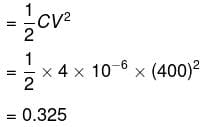This energy will be converted into heat in the resistor

31 Year NEET Previous Year Questions: Electrostatics Potential & Capacitance - Question 2

### A hollow metal sphere of radius 10 cm is chargedsuch that the potential on its surface is 80 V. Thepotential at the centre of the sphere is 

Detailed Solution for 31 Year NEET Previous Year Questions: Electrostatics Potential & Capacitance - Question 2

Potential at the centre of the sphere
= potential on the surface = 80 V.

31 Year NEET Previous Year Questions: Electrostatics Potential & Capacitance - Question 3

### The four capacitors, each of 25μ F are connected as shown in fig. The dc voltmeter reads 200 V. The charge on each plate of capacitor is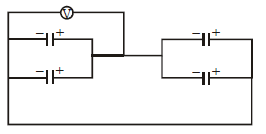Detailed Solution for 31 Year NEET Previous Year Questions: Electrostatics Potential & Capacitance - Question 3

Charge on each plate of each capacitor

Q =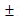CV =25 x10-6 x 200
=5 x10-3C

31 Year NEET Previous Year Questions: Electrostatics Potential & Capacitance - Question 4

If the potential of a capacitor having capacity 6μF is increased from 10 V to 20 V, then increase in its energy will be     

Detailed Solution for 31 Year NEET Previous Year Questions: Electrostatics Potential & Capacitance - Question 4

Capacitance of capacitor (C) = 6μF = 6 ×10–6 F; Initial potential (V1) = 10 V and final potential (V2) = 20 V.
The increase in energy (ΔU)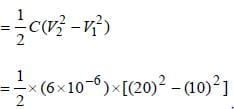= (3x10-6 )x300 = 9x10-4 J .

31 Year NEET Previous Year Questions: Electrostatics Potential & Capacitance - Question 5

A parallel plate condenser with oil between the plates (dielectric constant of oil K = 2) has a capacitance C. If the oil is removed, then capacitance of the capacitor becomes

Detailed Solution for 31 Year NEET Previous Year Questions: Electrostatics Potential & Capacitance - Question 5

When oil is placed between space of plates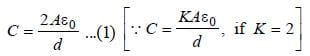When oil is removed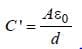... (2)

On comparing both equations, we get
C ' = C/2

31 Year NEET Previous Year Questions: Electrostatics Potential & Capacitance - Question 6

What is the effective capacitance between points X and Y?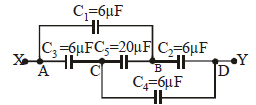Detailed Solution for 31 Year NEET Previous Year Questions: Electrostatics Potential & Capacitance - Question 6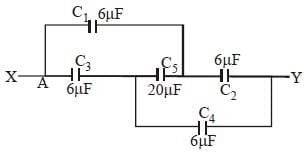Equivalent circuit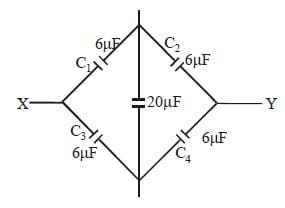Here,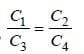Hence, no charge will flow through 20μF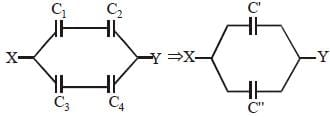C1 and C2 are in series, also C3 and C4 are in
series.
Hence, C' = 3 μF, C'' = 3 μF
C' and C'' are in parallel.
Hence net capacitance = C' + C'' = 3 + 3
= 6 μF

31 Year NEET Previous Year Questions: Electrostatics Potential & Capacitance - Question 7

A capacitor is charged to store an energy U.The charging battery is disconnected. Anidentical capacitor is now connected to the firstcapacitor in parallel. The energy in each of thecapacitor is 

Detailed Solution for 31 Year NEET Previous Year Questions: Electrostatics Potential & Capacitance - Question 7

Energy stored in a capacitor is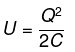As the battery is disconnected, total charge Q is shared equally by two capacitors.
So energy of each capacitor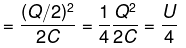31 Year NEET Previous Year Questions: Electrostatics Potential & Capacitance - Question 8

In a parallel plate capacitor, the distance between the plates is d and potential difference across the plates is V. Energy stored per unit volume between the plates of capacitor is

Detailed Solution for 31 Year NEET Previous Year Questions: Electrostatics Potential & Capacitance - Question 8

Energy stored per unit volume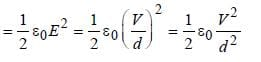(∵ E = V/d)

31 Year NEET Previous Year Questions: Electrostatics Potential & Capacitance - Question 9

The capacity of a parallel plate condenser is 10μF when the distance between its plates is 8cm. If the distance between the plates is reducedto 4 cm then the capacity of this parallel platecondenser will be 

Detailed Solution for 31 Year NEET Previous Year Questions: Electrostatics Potential & Capacitance - Question 9

C = 10 μF          d = 8 cm
C ' = ?                d ' = 4 cm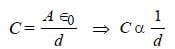If d is halved then C will be doubled.

Hence, C ' = 2C = 2 × 10μF = 20μF

31 Year NEET Previous Year Questions: Electrostatics Potential & Capacitance - Question 10

Energy stored in a capacitor is

Detailed Solution for 31 Year NEET Previous Year Questions: Electrostatics Potential & Capacitance - Question 10

Energy stored in capacitor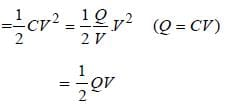31 Year NEET Previous Year Questions: Electrostatics Potential & Capacitance - Question 11

A capacitor C1 is charged to a potential difference V. The charging battery is then removed and the capacitor is connected to an uncharged capacitor C2. The potential difference across the combination is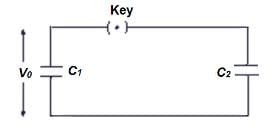Detailed Solution for 31 Year NEET Previous Year Questions: Electrostatics Potential & Capacitance - Question 11

Charge Q = C1V
Total capacity of combination (parallel)

C = C1+ C2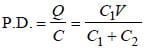31 Year NEET Previous Year Questions: Electrostatics Potential & Capacitance - Question 12

A solid spherical conductor is given a charge. The electrostatic potential of the conductor is      

Detailed Solution for 31 Year NEET Previous Year Questions: Electrostatics Potential & Capacitance - Question 12

Electric potential is constant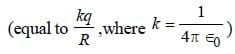within or on the surface of conductor.

31 Year NEET Previous Year Questions: Electrostatics Potential & Capacitance - Question 13

Each corner of a cube of side l has a negative charge, –q. The electrostatic potential energy of a charge q at the centre of the cube is    

Detailed Solution for 31 Year NEET Previous Year Questions: Electrostatics Potential & Capacitance - Question 13

Length of body diagonal =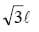∴ Distance of centre of cube from each
corner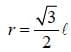P.E at centre
= 8 × Potential Energy due to A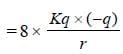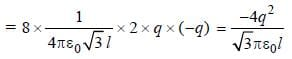31 Year NEET Previous Year Questions: Electrostatics Potential & Capacitance - Question 14

Three capacitors each of capacity 4μF are to be connected in such a way that the effective capacitance is 6 μF. This can be done by       

Detailed Solution for 31 Year NEET Previous Year Questions: Electrostatics Potential & Capacitance - Question 14

For series,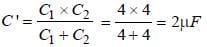For parallel, Ceq = C '+C3 = 2 + 4 = 6μF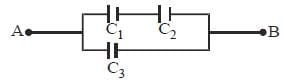31 Year NEET Previous Year Questions: Electrostatics Potential & Capacitance - Question 15

An electric dipole has the magnitude of its chargeas q and its dipole moment is p. It is placed inuniform electric field E. If its dipole moment is along the direction of the field, the force on itand its potential energy are respectively               

Detailed Solution for 31 Year NEET Previous Year Questions: Electrostatics Potential & Capacitance - Question 15

When the dipole is in the direction of field then net force is qE + (–qE) = 0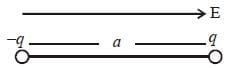and its potential energy is minimum
= – P.E. = –qaE

31 Year NEET Previous Year Questions: Electrostatics Potential & Capacitance - Question 16

A bullet of mass 2 g is having a charge of 2μC.Through what potential difference must it beaccelerated, starting from rest, to acquire a speedof 10 m/s?          

Detailed Solution for 31 Year NEET Previous Year Questions: Electrostatics Potential & Capacitance - Question 16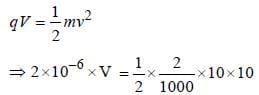∴ V = 50 kV

31 Year NEET Previous Year Questions: Electrostatics Potential & Capacitance - Question 17

As per the diagram, a point charge +q is placed at the origin O. Work done in taking another point charge –Q from the point A [coordinates (0, a)] to another point B [coordinates (a, 0)] along the straight path AB is: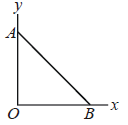Detailed Solution for 31 Year NEET Previous Year Questions: Electrostatics Potential & Capacitance - Question 17

We know that potential energy of two charge system is given by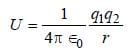According to question,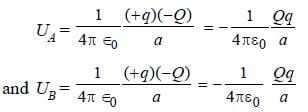ΔU= UB–UA = 0
We know that for conservative force,
W = –ΔU = 0

31 Year NEET Previous Year Questions: Electrostatics Potential & Capacitance - Question 18

Two charges q1 and q2 are placed 30 cm apart, as shown in the figure. A third charge q3 is moved along the arc of a circle of radius 40 cm from C to D. The change in the potential energy of the system is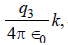where k is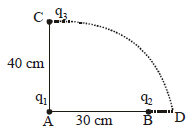Detailed Solution for 31 Year NEET Previous Year Questions: Electrostatics Potential & Capacitance - Question 18

We know that potential energy of discrete system of charges is given by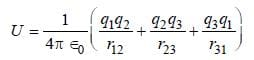According to question,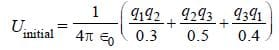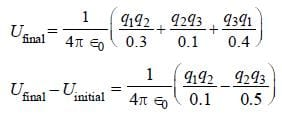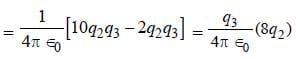31 Year NEET Previous Year Questions: Electrostatics Potential & Capacitance - Question 19

A network of four capacitors of capacity equal to C1 = C, C2 = 2C, C3 = 3C and C4 = 4C are conducted to a battery as shown in the figure. The ratio of the charges on C2 and C4 is: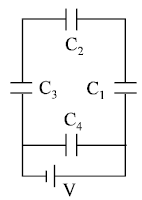Detailed Solution for 31 Year NEET Previous Year Questions: Electrostatics Potential & Capacitance - Question 19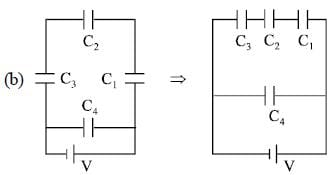Equivalent capacitance for three capacitors (C1, C2 & C3) in series is given by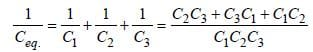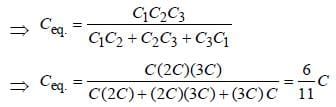⇒ Charge on capacitors (C1, C2 & C3) in series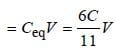Charge on capacitor C4 = C4V = 4C V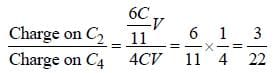31 Year NEET Previous Year Questions: Electrostatics Potential & Capacitance - Question 20

A parallel plate air capacitor is charged to apotential difference of V volts. After disconnecting the charging battery the distance between the plates of the capacitor is increased using an insulating handle. As a result the potential difference between the plates    

Detailed Solution for 31 Year NEET Previous Year Questions: Electrostatics Potential & Capacitance - Question 20

If we increase the distance between the plates its capacity decreases resulting in higher potential as we know Q = CV. Since Q is constant (battery has been disconnected), on decreasing C, V will increase

31 Year NEET Previous Year Questions: Electrostatics Potential & Capacitance - Question 21

Charges +q and –q are placed at points A and B respectively which are a distance 2L apart, C is the midpoint between A and B. The work done in moving a charge +Q along the semicircle CRD is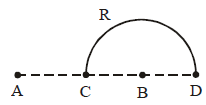Detailed Solution for 31 Year NEET Previous Year Questions: Electrostatics Potential & Capacitance - Question 21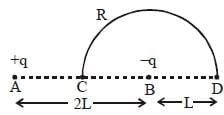Potential at C = VC = 0
Potential at D = VD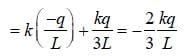Potential difference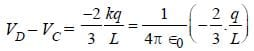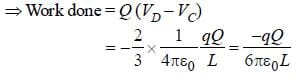31 Year NEET Previous Year Questions: Electrostatics Potential & Capacitance - Question 22

Two condensers, one of capacity C and other of capacity C/2 are connected to a V-volt battery, as shown.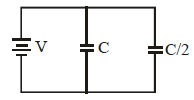The work done in charging fully both the  condensers is

Detailed Solution for 31 Year NEET Previous Year Questions: Electrostatics Potential & Capacitance - Question 22

Work done = Change in energy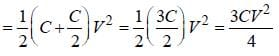31 Year NEET Previous Year Questions: Electrostatics Potential & Capacitance - Question 23

The electric potential at a point in free space due to a charge Q coulomb is Q × 1011 volts. The electric field at that point is 

Detailed Solution for 31 Year NEET Previous Year Questions: Electrostatics Potential & Capacitance - Question 23

Given that, V = Q × 1011 volts
Electric potential at point is given by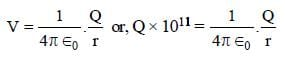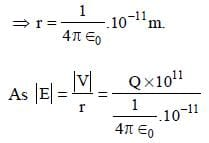= 4π∈0 Q x 1022 volt m-1

31 Year NEET Previous Year Questions: Electrostatics Potential & Capacitance - Question 24

The energy required to charge a parallel plate condenser of plate separation d and plate area of cross-section A such that the uniform electric field between the plates is E, is 

Detailed Solution for 31 Year NEET Previous Year Questions: Electrostatics Potential & Capacitance - Question 24

The energy required to charge a parallel
plate condenser is given by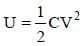As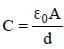and V = E.d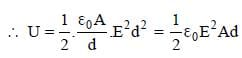31 Year NEET Previous Year Questions: Electrostatics Potential & Capacitance - Question 25

Three concentric spherical shells have radii a, b and c (a < b < c) and have surface charge densities σ , – σ and σ respectively. If VA, VB and VC denotes the potentials of the three shells, then for c = a + b, we have 

Detailed Solution for 31 Year NEET Previous Year Questions: Electrostatics Potential & Capacitance - Question 25

c = a + b.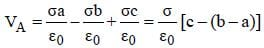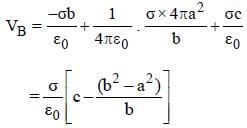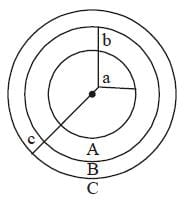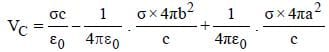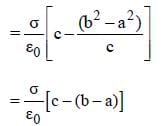VA = VC ≠ VB

31 Year NEET Previous Year Questions: Electrostatics Potential & Capacitance - Question 26

Three capacitors each of capacitance C and of breakdown voltage V are joined in series. The capacitance and breakdown voltage of the combination will be        

Detailed Solution for 31 Year NEET Previous Year Questions: Electrostatics Potential & Capacitance - Question 26

In series combination of capacitors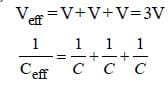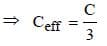Thus, the capacitance and breakdown
voltage of the combination will be C/3 and 3V

31 Year NEET Previous Year Questions: Electrostatics Potential & Capacitance - Question 27

The electric potential at a point (x, y, z) is given by V = – x2y – xz3 + 4. The electric field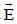at that point is   

Detailed Solution for 31 Year NEET Previous Year Questions: Electrostatics Potential & Capacitance - Question 27

The electric field at a point is equal to
negative of potential gradient at that point.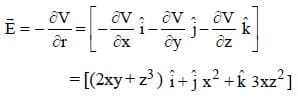31 Year NEET Previous Year Questions: Electrostatics Potential & Capacitance - Question 28

A series combination of n1 capacitors, each of value C1, is charged by a source of potential difference 4 V. When another parallel combination of n2 capacitors, each of value C2, is charged by a source of potential difference V, it has the same (total) energy stored in it, as the first combination has. The value of C2 , in terms of C1, is then   

Detailed Solution for 31 Year NEET Previous Year Questions: Electrostatics Potential & Capacitance - Question 28

In series,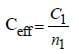∴ Energy stored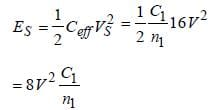In parallel, Ceff = n2 C2

∴ Energy stired ,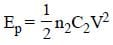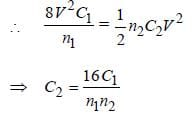31 Year NEET Previous Year Questions: Electrostatics Potential & Capacitance - Question 29

A condenser of capacity C is charged to a potential difference of V1. The plates of the condenser are then connected to an ideal inductor of inductance L. The current through the inductor when the potential difference across the condenser reduces to V2 is

Detailed Solution for 31 Year NEET Previous Year Questions: Electrostatics Potential & Capacitance - Question 29

q = CV1 cos ωt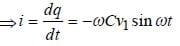Also,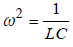and V = V1 cosωt

At t = t1 , V = V2 and i = -ωCV1 sinωt1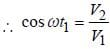(–ve sign gives direction)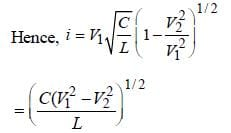31 Year NEET Previous Year Questions: Electrostatics Potential & Capacitance - Question 30

Two parallel metal plates having charges + Qand –Q face each other ata certain distance between them. If the plaves are now dipped inkerosene oil tank, the electric field between the plates will

Detailed Solution for 31 Year NEET Previous Year Questions: Electrostatics Potential & Capacitance - Question 30

Electric field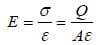ε of kerosine oil is more than that of air.
As ε increases, E decreases

31 Year NEET Previous Year Questions: Electrostatics Potential & Capacitance - Question 31

A parallel plate condenser has a uniform electric field E(V/m) in the space between the plates. If the distance between the plates is d(m) and area of each plate is A(m2) the energy (joules) stored in the condenser is 

Detailed Solution for 31 Year NEET Previous Year Questions: Electrostatics Potential & Capacitance - Question 31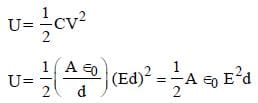31 Year NEET Previous Year Questions: Electrostatics Potential & Capacitance - Question 32

Four electric charges +q, +q, –q and – q are placed at the corners of a square of side 2L (see figure). The electric potential at point A, midway between the two charges +q and +q, is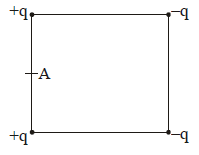Detailed Solution for 31 Year NEET Previous Year Questions: Electrostatics Potential & Capacitance - Question 32

Distance of point A from the two +q
charges = L.
Distance of point A from the two –q
charges =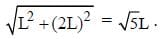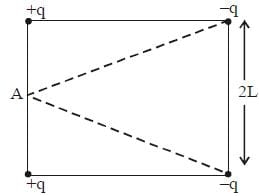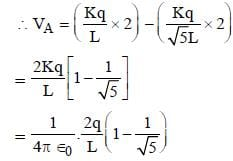31 Year NEET Previous Year Questions: Electrostatics Potential & Capacitance - Question 33

The potential energy of particle in a force field is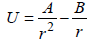, where A and B are positive constants and r is the distance of particle from the centre of the field. For stable equilibrium, the distance of the particle is : 

Detailed Solution for 31 Year NEET Previous Year Questions: Electrostatics Potential & Capacitance - Question 33

for equilibrium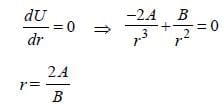for stable equilibrium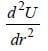should be positive for the value of r.

here,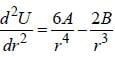is +ve value for r = 2A/B so

31 Year NEET Previous Year Questions: Electrostatics Potential & Capacitance - Question 34

Four point charges –Q, –q, 2q and 2Q are placed, one at each corner of the square. The relation between Q and q for which the potential at the centre of the square is zero is : 

Detailed Solution for 31 Year NEET Previous Year Questions: Electrostatics Potential & Capacitance - Question 34

Let the side length of square be 'a' then
potential at centre O is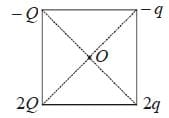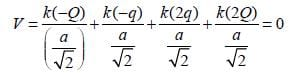(given)

= – Q – q + 2q + 2Q = 0 = Q + q = 0
= Q = – q

31 Year NEET Previous Year Questions: Electrostatics Potential & Capacitance - Question 35

A parallel plate capacitor has a uniform electric field E in the space between the plates. If the distance between the plates is d and area of each plate is A, the energy stored in the capacitor is :     [2012M]

Detailed Solution for 31 Year NEET Previous Year Questions: Electrostatics Potential & Capacitance - Question 35

The energy stored by a capacitor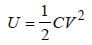...(1)

V is the p.d. between two plates of the
capacitor.
The capacitance of the parallel plate
capacitor
V = E.d.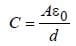Substituting the value of C in equation (i)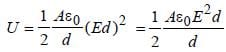31 Year NEET Previous Year Questions: Electrostatics Potential & Capacitance - Question 36

A, B and C are three points in a uniform electric field. The electric potential is [NEET 2013]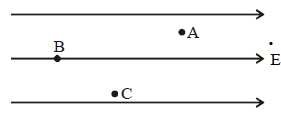Detailed Solution for 31 Year NEET Previous Year Questions: Electrostatics Potential & Capacitance - Question 36

Potential at B, VB is maximum
VB > VC > VA
As in the direction of electric field
potential decreases.

## Physics Class 12

157 videos|452 docs|213 tests
 Use Code STAYHOME200 and get INR 200 additional OFF Use Coupon Code
Information about 31 Year NEET Previous Year Questions: Electrostatics Potential & Capacitance Page
In this test you can find the Exam questions for 31 Year NEET Previous Year Questions: Electrostatics Potential & Capacitance solved & explained in the simplest way possible. Besides giving Questions and answers for 31 Year NEET Previous Year Questions: Electrostatics Potential & Capacitance, EduRev gives you an ample number of Online tests for practice

## Physics Class 12

157 videos|452 docs|213 tests

### How to Prepare for NEET

Read our guide to prepare for NEET which is created by Toppers & the best Teachers